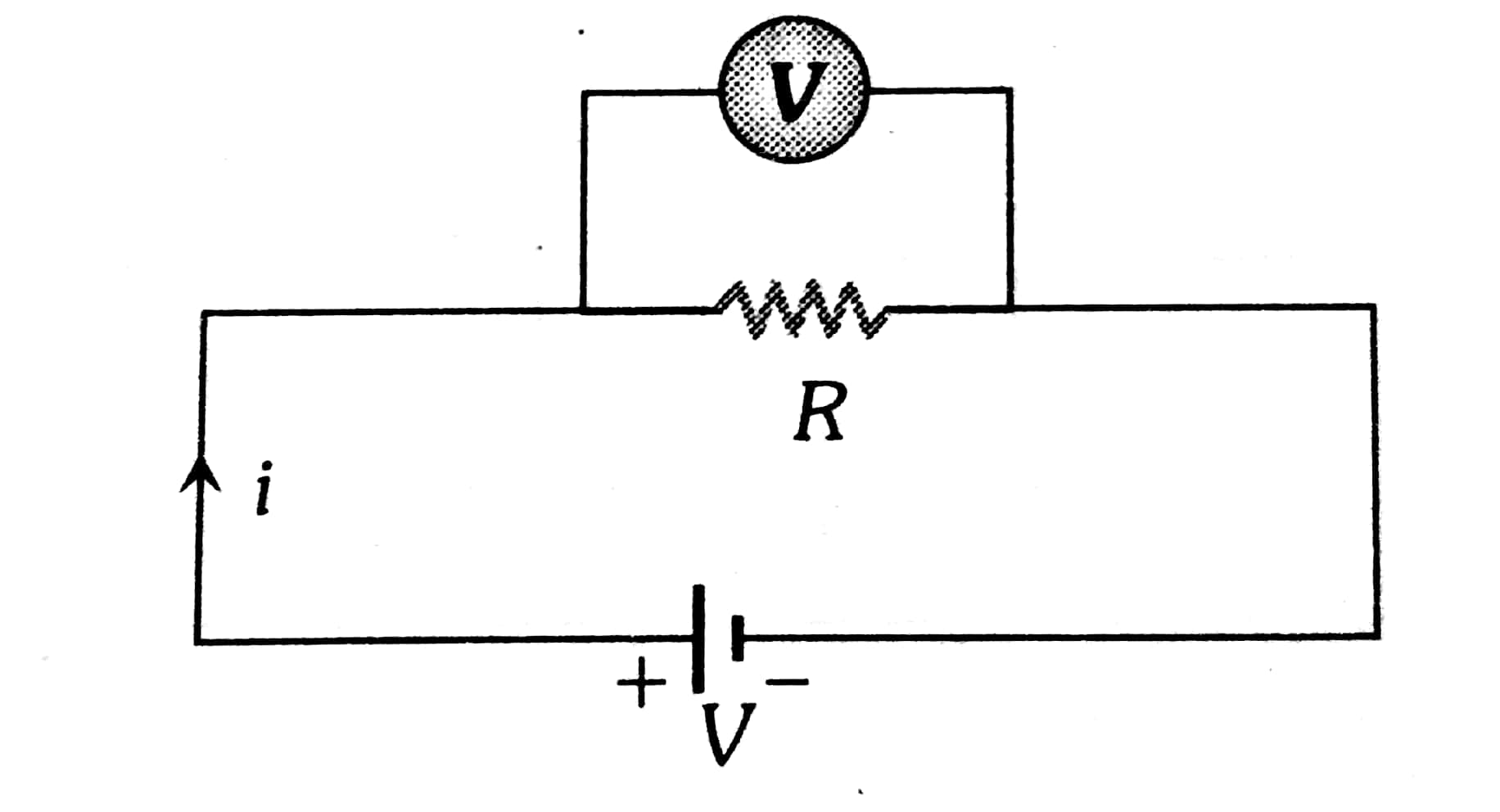#### Two voltameters, one of copper and another of silver, are joined in parallel. When a total chargeflows through the voltmeters, equal amount of metals are deposited. If the electrochemical equivalents of copper and silver arerespectively the charge which flows through the silver voltameter is Option 1)Option 2)Option 3)Option 4)As we learnt in

Voltameter -

It is a device used to measure Potential difference and is always put in parallel with circuit element

- whereinThe voltameters joined are parallel mass depositedOption 1)This option is incorrect.

Option 2)This option is incorrect.

Option 3)This option is incorrect.

Option 4)This option is correct.

#### prateek